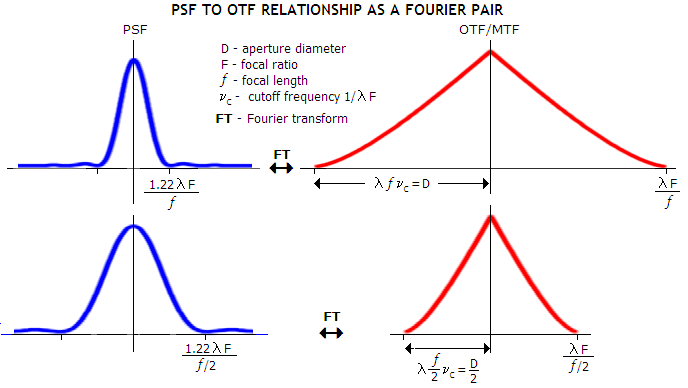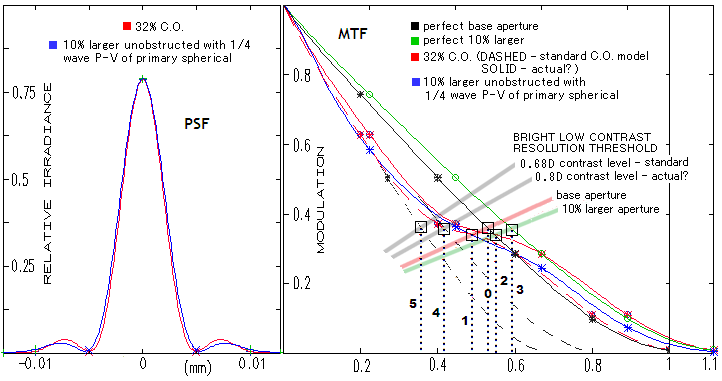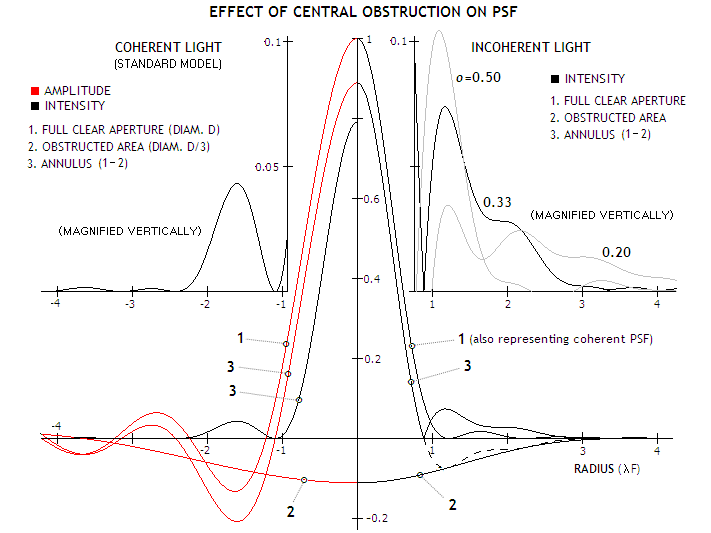telescopeѲptics.net          ▪▪▪▪                                             CONTENTS

7.1.2. Telescope central obstruction: size criteria

7.1.1. CENTRAL OBSTRUCTION EFFECT: THEORETICAL INCONSISTENCIES?

INCONSISTENCIES

1. According to the theory, annular aperture has MTF cutoff frequency identical to that of circular aperture of the same diameter. Since central obstruction (CO) results in smaller central maxima, the cutoff frequency (i.e. limiting MTF resolution) should be proportionally smaller.

The usual way of obtaining OTF/MTF is from the normalized exit pupil autocorrelation, where the integration area for the contrast transfer for aberration-free aperture is graphically presented as the overlap - normalized to the circle area - of two identical circles of the diameter equaling λfνc, where λ is the wavelength, f the focal length and νc the cutoff frequency. With νc=1/λF, F being the focal ratio, the center separation of these two circles at the cutoff frequency equals the circle (i.e. pupil) diameter. The same formalism is used to obtain OTF/MTF (for aberration-free aperture the phase OTF component is unity, and OTF reduces to MTF) of annular aperture, except that the overlap area excludes the area of central obstruction, and overlap is normalized to the annulus area (FIG. 104). Hence, the formalism results in the cutoff frequency for obstructed aperture independent of the size of obstruction, and equal to that of clear circular aperture, 1/λF.

While this formalism should accurately describe contrast transfer between zero and cutoff frequency for annular aperture, it is not appropriate for determining the cutoff frequency. The reason is that annular pupil produces diffraction pattern reduced in size with respect to that of clear aperture of the same diameter - in effect, a pattern nearly identical to one produced by a larger clear aperture with spherical aberration, in which the level of aberration does not cause change in cutoff frequency. In other words, it is physically impossible that a near-identical impulse response (PSF) produces two different MTF cutoff frequencies.

This conclusion is consistent with the relation between PSF and OTF, which are a Fourier pair, implying that any given PSF will produce a single corresponding OTF. Since the annular aperture PSF is narrower than that of a clear aperture of the same diameter, its OTF will be wider, i.e. will expand toward higher frequencies. Plots below illustrate the Fourier transform relationship between angular size of the PSF (first minima 1.22λ/D radians) and the extent of OTF frequency spectrum (linear cutoff period λF and frequency 1/λF, angular cutoff period λ/D period and angular cutoff frequency D/λ) for circular pupil of diameter D (top) and for half as large aperture (bottom) of identical focal ratio.Properties of the ring structure depend on pupil transmission properties but OTF width depends only on the first minima radius. In general, reduction of transmission in the central pupil area increases the magnitude and/or size of (primarily) the 1st bright ring and reduces the central maxima radius, while reduction in the outer area suppresses the rings but increases the maxima radius; aberrations effectively alter transmission over pupil as well, so they also affect the central maxima radius and the rings' radii and magnitude.

Follows an example of the obstructed aperture PSF and its properties, indicating the same conclusion, i.e. that annular aberration-free aperture must have higher MTF cutoff frequency than circular aperture of the same diameter. Raytrace was done with D=152mm f/8.18 mirror in polychromatic light (25 wavelengths from 440nm to 680nm, weighted for photopic eye sensitivity). Base (obstructed) aperture diameter was 152mm, and the aberrated by 10% larger.

LEFT: PSF of a clear aberration-free aperture of diameter D with 0.33D central obstruction (red) nearly coincides with the PSF of the 10% larger (linearly, 1.1D diameter) clear aperture with λ/4 wave P-V of primary spherical (blue). Central maxima of the obstructed aperture - reduced by about 10% in diameter due to the effect of central obstruction - is nearly identical to that of the larger aberrated clear aperture in monochromatic light; however, raytrace in polychromatic light indicates it is still some 7% smaller (despite the pSF maxima of the aberrated aperture being also slightly smaller, due to the aberration). Since the focal length is constant, the PSF maxima - including FWHM - in the smaller obstructed aperture is actually smaller angularly than in the larger aperture. Yet, according to the standard MTF formalism, the smaller obstructed aperture will have 10% lower cutoff frequency, given as D/λ (cycles per radian; related to the linear resolution as D/λƒ=1/λF lines per mm, ƒ being the focal length and F the focal ratio), i.e. as much worse limiting MTF resolution. Evidently, there is no basis for such a difference in their respective PSFs. In fact, the plane physical parameters directly imply that the smaller obstructed aperture will actually have higher cutoff frequency than the larger one.MTF cutoff frequency does not change for primary spherical aberration up to 1/2 wave P-V, and somewhat larger, which implies that more energy outside the Airy disc shouldn't affect cutoff frequency for larger CO as well. As mentioned above, the main determinant is the diameter of central maxima. Since the reduction of the PSF angular size in the obstructed aperture is well approximated by 1-o2 (somewhat optimistic for obstructions significantly larger than D/3), angular size of its central maxima - and its MTF cutoff frequency - are better approximated as those corresponding to an aperture larger by a factor of
D/(1-
o2). Consequently, contrast transfer at any normalized spatial frequency ν of unobstructed aperture nearly corresponds to (1-o2)ν frequency of the obstructed aperture.

In effect, the obstructed aperture's PSF becomes comparable to that of a clear aperture larger by a factor 1/(1-ο2) having its Strehl reduced to (1-ο2)2 by spherical aberration. Since the central maxima also shrinks with the increase in primary spherical aberration, although significantly less than with c. obstruction - nearly 3% with λ/4 wavefront error, and nearly 9% with λ/2 error - it will partly offset the larger rate of obstructed maxima diameter reduction in polychromatic light.

On the graph, we see that even with the standard MTF formalism the 1-o "rule" is, for this obstruction size - placing its contrast level at about 0.68D aperture level - valid only for the lower third of the frequency range. Contrast rapidly recovers toward higher frequencies: it is at the level of 0.75D already at the 0.4 frequency, and at about 0.9D level at the bright low-contrast cutoff at ~0.55 frequency. For bright low contrast details, contrast dropping to 0.68D level would cause resolution threshold to also drop by a third (5 vs. 0), but the threshold is even in the standard MTF model better, about 27% lower than for perfect base aperture. If going by the contrast level projected from the likely obstructed plot at about 0.8D, the threshold is worsened by little over 20% vs. base unobstructed aperture (4 vs. 0). But at the actual point of intersection for the likely obstructed aperture plot, the threshold is 4-5% above the base aperture (2 vs. 0). It is about 7% smaller than the threshold for 10% larger aperture (3) when aberration-free, and a few percent larger when it has 1/4 wave of primary spherical aberration.

Raytrace gives imilar results for other central obstruction sizes. For instance, the PSF of 20% larger linear aperture aberrated by λ/2 wave P-V wavefront error of spherical aberration is nearly identical to the PSF of the smaller aperture with 0.6D CO (the only difference being in mainly offsetting energy content in the 2nd and 3rd bright ring). Here (1-ο2)2 gives as the PSF-equilizing Strehl value of 0.41, with the actual Strehl for this amount of spherical aberration being 0.38. And, yet again, despite the two nearly identical PSFs, the standard MTF formalism tells us that the larger aberrated aperture has 20% higher cutoff frequency than the smaller obstructed one.

It should be noted that FWHM shrinks due to CO at somewhat slower rate than the 1st minima radius for obstructions ~D/4 and larger. Specifically, the FWHM-deduced aperture enlargement is smaller from about 30% at 0.3D to about 40% at 0.5D CO. FWHM is more relevant to diffraction resolution than the 1st minima radius, but in polychromatic light it would be mainly offset by the larger rate of obstruction-induced central minima reduction, implied by raytrace.

Better contrast transfer of obstructed apertures than what the standard MTF formalism indicates would also help explain why the popular 1-o "rule" - based on that formalism - seems to be at least roughly working, even if it completely neglects significant differences in the total aberration level between smaller unobstructed and larger obstructed apertures (seeing, thermals, inherent aberrations, alignment, surface/coating quality, etc.).

2. Theory models CO effect on a near-monochromatic, practically coherent point-source. The light we observe is polychromatic, thus closer to incoherent (temporally). In addition, light from practically all objects extended beyond point-like is also spatially incoherent. Since coherent and incoherent light have different dependencies on the changes in pupil transmission, CO effect will also be different. This is not inconsistency, rather insufficiency in the theoretical model, which does not address this real-world scenario. An attempt is made here to get indications of what the CO effect is in incoherent light, based on the very basic differences in wave interference of coherent vs. incoherent light.

While PSF has identical form for coherent and incoherent light, the actual energy and the way it is generated differ between the two. Light gathering power of astronomical telescopes, given transmission, changes with the area of aperture. So, doubling the aperture quadruples its area, hence also light gathering power and the actual peak diffraction intensity (in terms of magnitudes, every doubling in linear aperture gains 1.5 magnitude). This is because incoherent waves add up their energy individually, as a sum of their individual squared amplitudes. This is what makes incoherent light linear in intensity. On the other hand, coherent waves, due to their strong phase correlation, generate energy more efficiently: their individual amplitudes add up to a complex amplitude, which is then squared for energy. That makes them linear in amplitude, and quadratic in intensity (energy). In other words, quadrupling pupil area quadruples the complex amplitude, and increases the energy 16-fold.

These different dependencies of the energy on the pupil area imply that any given central obstruction - or any obstruction, for that matter - will have different effect in coherent vs. incoherent light. In the standard theory of diffraction in annular aperture, diffraction peak (normalized to 1 for clear aperture) is given by the relative annulus area squared - i.e. by (1-o2)2, the limit of I=[2J1(x)/x-o22J1(ox)/ox]2 for x=πr=0 (see Eq. c). This is consistent with coherent, but not with incoherent light. For the latter, being linear in intensity, the appropriate general expression would be I=[2J1(x)/x]2-o2[2J1(ox)/ox]2, with the energy at every image point being a sum of the squared individual wave amplitudes, less those from the obstructed area. In terms of amplitudes, it is given as I'(r)=ΣAt2-ΣAot2, which for r=0 (central point of the diffraction pattern) is I'(0)=ΣAt2-Σ(oAt)2. Hence, the normalized peak intensity here is reduced in proportion to 1-o2.

Since deducting the CO area amplitude contribution and squaring the difference (in coherent light) produces different result than deducting energy from this area directly (in incoherent light), the entire annular aperture PSF will differ for the two, not only its central intensity. Considering that PSF-defined intensity distribution for point-source image determines contrast and resolution of extended objects as well, any significant difference in the annular PSF will imply significant difference in the effect of CO on overall performance.

Applying this method of deducting the contribution of the obstructed area from the contribution of the entire aperture area at every image point for coherent and incoherent  light in order to obtain the resulting PSF is shown below. Complex amplitude for the aperture of D/3 diameter is shown negative (in the central portion) because it is an inverted aperture, whose complex amplitude is effectively deducted from the full aperture complex amplitude. Since complex amplitude for full aperture has segments with negative values, the negative complex amplitude of the central obstruction area effectively adds up to it, making the absolute value of the complex amplitude - thus the resulting intensity as well - of the annulus higher for these segments (e.g. first bright ring), as shown on the intensity plot for coherent light.

With the intensity of incoherent light being a sum of squared individual wave amplitudes, they add up linearly to the intensity at every point of the point image, in the same proportion as squared amplitudes do with coherent light, and in an identical pattern of maximas and minimas. This implies that there are analogous constructive and destructive interference on the level of intensity combining as well, the difference being that the intensities always remain positive in sign. Constructive contribution would max out in the central peak, drop to zero at the first minima, to re-emerge as constructive residual in the series of decaying subsidiary maximas. So for instance, taking out wave contribution from the obstructed pupil area would result in the intensity level at the first minima to rise to the level of the intensity level of the obscured area PSF at that point (since it was brought to zero by an equal in magnitude but destructive intensity contribution from the annulus area). Intensity level at the 2nd maxima of unobstructed aperture will, with introduction of central obstruction, become the difference between intensity of the obstructed area PSF and clear aperture PSF at that point, and so on. In effect, the resulting intensity distribution is obtained by deducting intensity distribution that would result from wave contribution from the obstructed area from the clear aperture's intensity distribution - but with resulting intensity remaining always positive in sign.The resulting plots show that the obstructed PSF in incoherent light, while having less depressed central maxima, has more energy transferred to the rings area, with the 2nd and 3rd bright ring merging. Note that normalizing both obstructed (intensity) PSFs, coherent and incoherent, to 1 would result in the incoherent obstructed PSF to shrink vs. coherent by a factor of 0.89. Still, the second maxima in the former remains significantly larger and stronger.

There is no appreciable difference in the central maxima shape for coherent vs. incoherent obstructed PSF normalized to 1 - including FWHM - until the last third, or so, of the (coherent PSF') radius, when the latter starts dropping in intensity faster, falling to the first minima at a radius about 20% shorter vs. coherent obstructed PSF (and about 28% shorter vs. unobstructed PSF's central maxima). Thus the difference in volume between the first two is significantly smaller than what the radius alone indicates.

In all, this coarse modeling based on the very elementary difference in interference for coherent vs. incoherent waves indicates that the effect of central obstruction is different, and probably larger with the latter, with the most significant differences being yet smaller central maxima and 2nd bright ring tending to merge with the 3rd at mid-to-small obstruction sizes. As the magnified rings for D/2 and D/5 obstructions show, there would be significantly less of a difference in the amount of energy displaced to the rings area than with coherent light, with smaller obstructions brightening the ring area less but in a wider radius, and larger obstructions causing more of a brightening, but over narrower range, closer to the central maxima.

Should be noted that incoherent light in this model is practically near-monochromatic, as the presence of different wavelengths (polychromatic light) would induce distortions to the intensity distribution due to the PSF scale varying with the wavelength (judging on the polychromatic PSF, those distortions would be relatively small, due to both, typical object emission and eye sensitivity tending to peak in a relatively narrow spectral range).

The actual light from astronomical objects is neither incoherent, nor coherent. It is generally closer to incoherent (partially incoherent), so the actual CO effect would be, correspondingly, closer to that indicated by the incoherent model. While this modeling for the effect of central obstruction in incoherent light is, as already mentioned, very approximate, it is logical for it to be greater in coherent light, because taking out energy originating from the obstruction area at the level of amplitude has increasingly smaller effect going farther from the center - due to the squaring for intensity - than taking out intensity directly in incoherent light.

With the above in mind, the rest of this section will be based on the standard theoretical approach to the effect of central obstruction, assuming near monochromatic (coherent) point-source.

Commonly raised question with respect to central obstruction in a telescope is its maximum acceptable size. The answer necessarily vary somewhat with the particular instrument and its primary use.